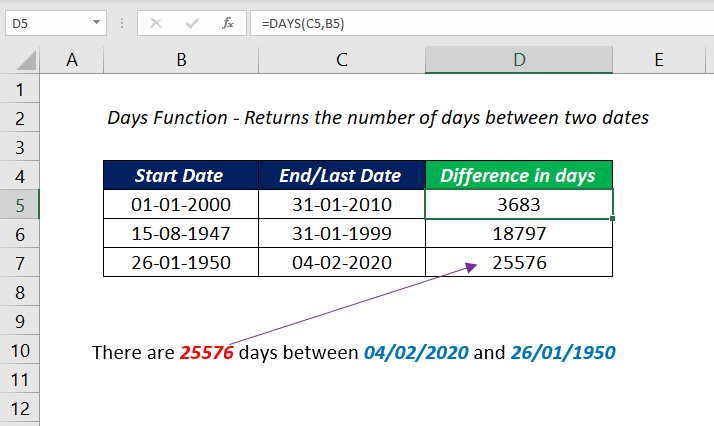# DAYS FunctionThe Excel DAYS function is a DATE & TIME function that can return the number of days between two dates.

Date and time

### Purpose

Find the number of days between two dates

### Return value

An integer representing the number of days

### Syntax

`=DAYS (end_date, start_date) `

### Arguments

end_date – The end date

start_date – The start date

### Examples

#### Number of days between two Dates (Direct Values)

The following formula will return the number of days between the two dates 01/01/2000 and 31/01/2018

`=DAYS("01/01/2000","31/01/2018")`

#### Number of days between two Dates (cell references)

The following formula will return the difference between the two dates in the cells C3 and B3.

`=DAYS(C3,B3)`